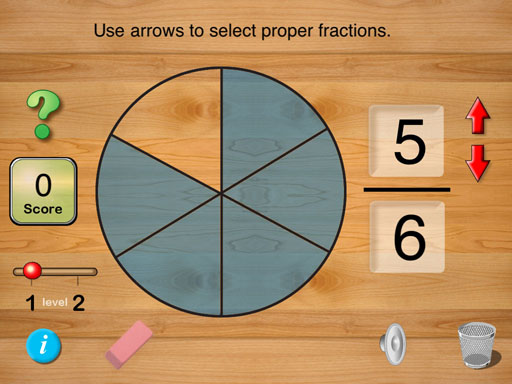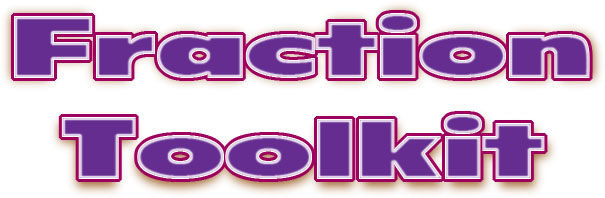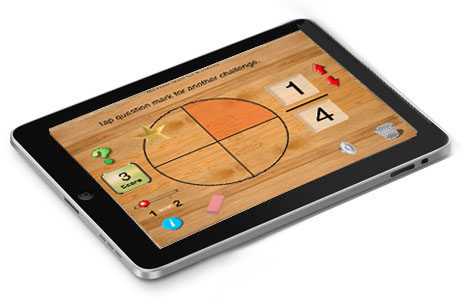Challenge your students to improve their math skills the fun way!Interactive Excitement and a Great Learning Experience
 Fraction Toolkit is used to learn about identifying and simplifying proper fractions. It has open-ended features that let student enter the numerator and denominator for a proper fraction and see a graphical representation of the fraction. The app has two levels. At level 1, by tapping the question mark the student is prompted to enter the numerator and denominator for a given graphical representation of a fraction. At level 2, when the question mark is tapped the student is challenged to enter a fraction in lowest terms. The app keeps track of student performance and increases the student's score each time a correct answer is given. A point is subtracted from the score when correct answers are given. Teachers can gauge a student's s performance by looking at how many points were scored during a given activity period.Special features make using Fraction Toolkitfun: 1. Tap arrows to define numerator and denominator. 2. Random problems are automatically generated. 3. Provide practice identifying and simplifying fractions. 4. Sound effects. 5. Available for iPad iOS 7 and 8.\$0.99